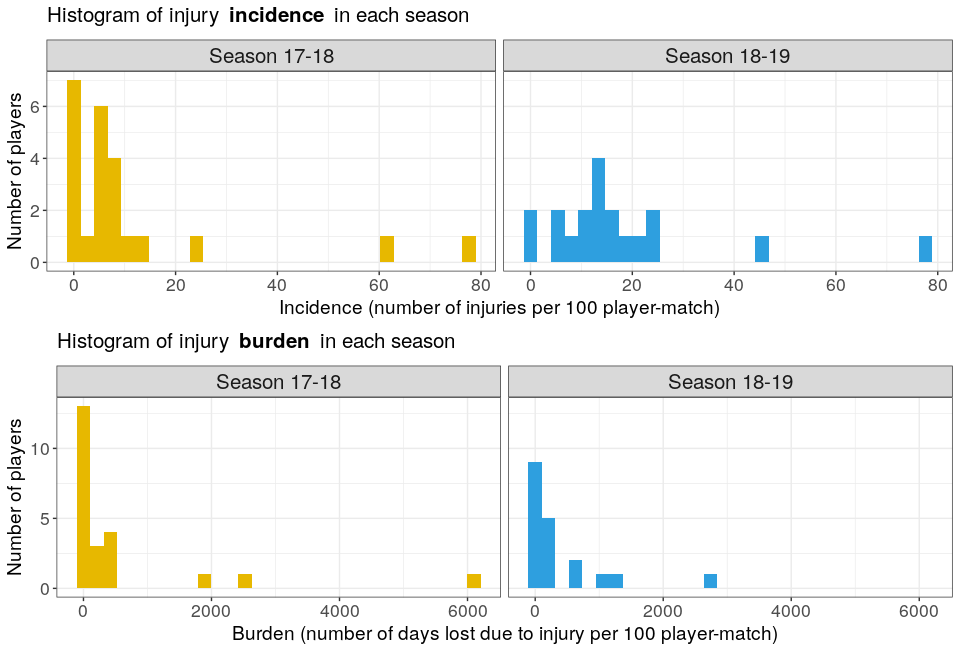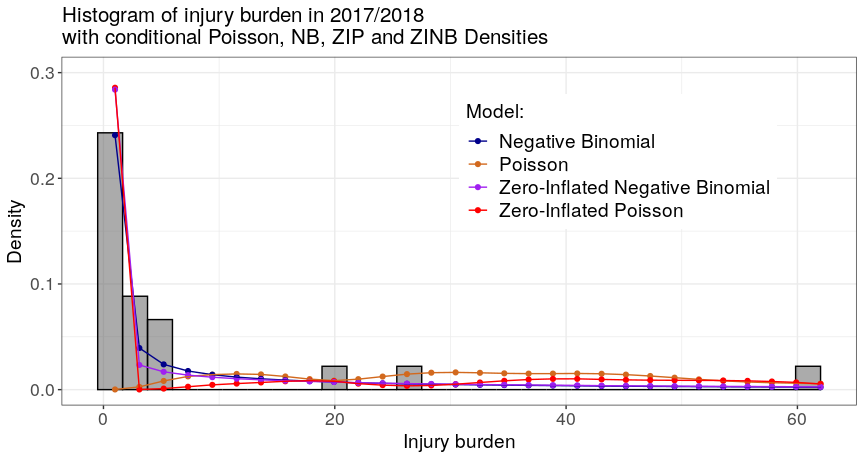# Model Sports Injuries as Counts

#### 2023-11-14

library(injurytools)
library(dplyr)
library(stringr)
library(tidyr)
library(lme4)
library(pscl)
# library(glmmTMB)
library(MASS)
library(ggplot2)
library(gridExtra)
library(knitr)

Example data: we continue exploring the cohort of Liverpool Football Club male’s first team players over two consecutive seasons, 2017-2018 and 2018-2019, scrapped from https://www.transfermarkt.com/ website1.

This article provides some modelling approaches when injuries are seen as count data.

First, the distribution of the count/rate variables is explored, e.g. number of injuries and number of days lost per exposure time. Afterwards, four different models are presented, that depending on the distribution of the data, will provide a better fit to them due to the distributional assumptions made by each of them.

# Exploring the distribution of injuries

We plot the histograms of the injury incidence and injury burden variables for each season.

See the code to prepare the data
## 17/18
df_exposures1718 <- prepare_exp(df_exposures0 =
raw_df_exposures |> filter(season == "17/18"),
player        = "player_name",
date          = "year",
time_expo     = "minutes_played") |>
mutate(seasonb = date2season(date))
df_injuries1718 <- prepare_inj(df_injuries0   =
raw_df_injuries |> filter(season == "17/18"),
player         = "player_name",
date_injured   = "from",
date_recovered = "until")
injd1718 <- prepare_all(data_exposures = df_exposures1718,
data_injuries  = df_injuries1718,
exp_unit = "matches_minutes")
## 18/19
df_exposures1819 <- prepare_exp(df_exposures0 =
raw_df_exposures |> filter(season == "18/19"),
player        = "player_name",
date          = "year",
time_expo     = "minutes_played") |>
mutate(seasonb = date2season(date))
df_injuries1819 <- prepare_inj(df_injuries0   =
raw_df_injuries |> filter(season == "18/19"),
player         = "player_name",
date_injured   = "from",
date_recovered = "until")
injd1819 <- prepare_all(data_exposures = df_exposures1819,
data_injuries  = df_injuries1819,
exp_unit = "matches_minutes")
## calculate injury summary statistics
injds1718 <- injsummary(injd1718, quiet = T)
injds1819 <- injsummary(injd1819, quiet = T)

injds1718p <- injds1718$playerwise injds1819p <- injds1819$playerwise

injdsp <- bind_rows("Season 17-18" = injds1718p,
"Season 18-19" = injds1819p,
.id = "season")
## plot
p1 <- ggplot(data = injdsp) +
geom_histogram(aes(x = injincidence, fill = season)) +
facet_wrap(~season) +
scale_fill_manual(name = "", values = c("#E7B800", "#2E9FDF")) +
ylab("Number of players") +
xlab("Incidence (number of injuries per 100 player-match)") +
ggtitle("Histogram of injury incidence in each season") +
theme(legend.position = "none")

p2 <- ggplot(data = injdsp) +
geom_histogram(aes(x = injburden, fill = season)) +
facet_wrap(~season) +
scale_fill_manual(name = "", values = c("#E7B800", "#2E9FDF")) +
ylab("Number of players") +
xlab("Burden (number of days lost due to injury per 100 player-match)") +
ggtitle("Histogram of injury burden in each season") +
theme(legend.position = "none")

grid.arrange(p1, p2, ncol = 1)
Code for further plot specifications
theme_counts <- theme(axis.text = element_text(size = rel(1.2)),
axis.title = element_text(size = rel(1.3)),
strip.text = element_text(size = rel(1.4)),
plot.title = element_text(size = rel(1.4)),
legend.text = element_text(size =  rel(1.3)),
legend.title = element_text(size = rel(1.3)))
p1 <- p1 + theme_counts
p2 <- p2 + theme_countsIn the following, we use injds1718p data.

# - Poisson (mixed) model

We merge into the injds1718p data frame the player-related information (i.e. positionb, age, assists, goals, yellows etc.) available in the raw_df_exposures data frame.

## 17/18
df_exposures1718 <- prepare_exp(df_exposures0 =
raw_df_exposures |> filter(season == "17/18"),
player        = "player_name",
date          = "year",
time_expo     = "minutes_played") |>
mutate(seasonb = date2season(date))

injds1718p <- injds1718p |>
mutate(seasonb = "2017/2018") |>
## join to have info, such as position, age, citizenship etc.
left_join(df_exposures1718, by = c("player" = "player",
"seasonb" = "seasonb")) |>
## create positionb column
## (so that the categories are: Attack, Defender, Goalkeeper and Midfield)
mutate(positionb = factor(str_split_i(position, "_", 1)))
## quit Goalkeepers
injds1718p <- dplyr::filter(injds1718p, positionb != "Goalkeeper") |>
droplevels()

To fit a Poisson regression model for injury incidence (considering positionb as a covariate), we do:

incidence_glm_pois <- glm(ninjuries ~ positionb, # + offset(log(totalexpo))
offset = log(totalexpo),
data = injds1718p,
family = poisson)

Or alternatively, we can use the glmmTMB::glmmTMB function:

# incidence_glm_pois2 <- glmmTMB(formula = ninjuries ~ foot,
#                                offset = log(totalexpo),
#                                family = poisson(), data = injds1718p)
# summary(incidence_glm_pois2)

Besides, if we have repeated measurements as in injdsp, we can fit a Mixed Model via:

Add more covariates to injdsp data frame
df_exposures <- prepare_exp(df_exposures0 = raw_df_exposures,
player        = "player_name",
date          = "year",
time_expo     = "minutes_played") |>
mutate(seasonb = date2season(date))

injdsp <- injdsp |>
mutate(seasonb = if_else(season == "Season 17-18", "2017/2018", "2018/2019")) |>
## join to have info, such as position, age, citizenship etc.
left_join(df_exposures, by = c("player" = "player",
"seasonb" = "seasonb")) |>
## create positionb column
## (so that the categories are: Attack, Defender, Goalkeeper and Midfield)
mutate(positionb = factor(str_split_i(position, "_", 1))) |>
droplevels()
incidence_glmm_pois <- glmer(formula = ninjuries ~ positionb + (1 | player),
offset = log(totalexpo),
data = injdsp,
family = poisson)
# incidence_glmm_pois2 <- glmmTMB::glmmTMB(formula = ninjuries ~ positionb + (1 | player),
#                                          offset = log(totalexpo),
#                                          data = injdsp,
#                                          family = poisson)

We can do the analogue for the burden, e.g.:

burden_glm_pois <- glm(ndayslost ~ positionb, offset = log(totalexpo), ## or ~ foot
data = injds1718p,
family = poisson)

As of now, lets stick with the model burden_glm_pois and lets interpret the model output.

summary(burden_glm_pois)
#>
#> Call:
#> glm(formula = ndayslost ~ positionb, family = poisson, data = injds1718p,
#>     offset = log(totalexpo))
#>
#> Coefficients:
#>                   Estimate Std. Error z value Pr(>|z|)
#> (Intercept)       -3.81936    0.06651 -57.422   <2e-16 ***
#> positionbDefender -0.03227    0.08893  -0.363    0.717
#> positionbMidfield  1.01561    0.07780  13.054   <2e-16 ***
#> ---
#> Signif. codes:  0 '***' 0.001 '**' 0.01 '*' 0.05 '.' 0.1 ' ' 1
#>
#> (Dispersion parameter for poisson family taken to be 1)
#>
#>     Null deviance: 2622.1  on 20  degrees of freedom
#> Residual deviance: 2327.1  on 18  degrees of freedom
#> AIC: 2417.3
#>
#> Number of Fisher Scoring iterations: 6

The estimated coefficients are:

cbind(estimate = exp(coef(burden_glm_pois)) * c(90*100, 1, 1),
exp(confint(burden_glm_pois)) * c(90*100, 1, 1)) |> # (to report per 100 player-matches)
kable()
#> Waiting for profiling to be done...
estimate 2.5 % 97.5 %
(Intercept) 197.4757300 172.8356815 224.351527
positionbDefender 0.9682499 0.8137691 1.153448
positionbMidfield 2.7610445 2.3746045 3.221913

# - Negative Binomial model

burden_glm_nb <- glm.nb(ndayslost ~ positionb + offset(log(totalexpo)),
data = injds1718p)
summary(burden_glm_nb)
#>
#> Call:
#> glm.nb(formula = ndayslost ~ positionb + offset(log(totalexpo)),
#>     data = injds1718p, init.theta = 0.2625149285, link = log)
#>
#> Coefficients:
#>                   Estimate Std. Error z value Pr(>|z|)
#> (Intercept)        -2.9829     0.7404  -4.029  5.6e-05 ***
#> positionbDefender  -0.8857     1.0139  -0.874    0.382
#> positionbMidfield   1.1416     1.0882   1.049    0.294
#> ---
#> Signif. codes:  0 '***' 0.001 '**' 0.01 '*' 0.05 '.' 0.1 ' ' 1
#>
#> (Dispersion parameter for Negative Binomial(0.2625) family taken to be 1)
#>
#>     Null deviance: 27.799  on 20  degrees of freedom
#> Residual deviance: 24.105  on 18  degrees of freedom
#> AIC: 200.83
#>
#> Number of Fisher Scoring iterations: 1
#>
#>
#>               Theta:  0.2625
#>           Std. Err.:  0.0804
#>
#>  2 x log-likelihood:  -192.8270

# - Zero-inflated Poisson model

burden_zinfpois <- zeroinfl(ndayslost ~ positionb | positionb,
offset = log(totalexpo),
data = injds1718p,
dist = "poisson",
trace = FALSE, EM = FALSE)
## Or:
# burden_zinfpois <- glmmTMB::glmmTMB(formula = ndayslost ~ 1 +  positionb,
#                                     offset = log(totalexpo),
#                                     ziformula = ~ 1 + positionb,
#                                     data = injds1718p,
#                                     family = poisson)
summary(burden_zinfpois)
#>
#> Call:
#> zeroinfl(formula = ndayslost ~ positionb | positionb, data = injds1718p,
#>     offset = log(totalexpo), dist = "poisson", link = "logit", trace = FALSE,
#>     EM = FALSE)
#>
#> Pearson residuals:
#>    Min     1Q Median     3Q    Max
#> -2.186 -1.385 -1.028  1.840 19.448
#>
#> Count model coefficients (poisson with log link):
#>                   Estimate Std. Error z value Pr(>|z|)
#> (Intercept)       -3.38576    0.06652 -50.897   <2e-16 ***
#> positionbDefender -0.21247    0.08894  -2.389   0.0169 *
#> positionbMidfield  0.77310    0.07781   9.936   <2e-16 ***
#>
#> Zero-inflation model coefficients (binomial with logit link):
#>                   Estimate Std. Error z value Pr(>|z|)
#> (Intercept)        -0.2877     0.7638  -0.377    0.706
#> positionbDefender  -0.8109     1.1181  -0.725    0.468
#> positionbMidfield  -1.3218     1.3354  -0.990    0.322
#> ---
#> Signif. codes:  0 '***' 0.001 '**' 0.01 '*' 0.05 '.' 0.1 ' ' 1
#>
#> Number of iterations in BFGS optimization: 1
#> Log-likelihood: -929.5 on 6 Df

# - Zero-inflated Negative Binomial model

burden_zinfnb <- zeroinfl(ndayslost ~ positionb | positionb,
offset = log(totalexpo),
data = injds1718p,
dist = "negbin",
trace = FALSE, EM = FALSE)
## Or:
# burden_zinfnb <- glmmTMB::glmmTMB(ndayslost ~ 1 + positionb, offset = log(totalexpo),
#                                      ziformula = ~ 1 + positionb,
#                                      data = injds1718p,
#                                      family = nbinom2)
summary(burden_zinfnb)
#>
#> Call:
#> zeroinfl(formula = ndayslost ~ positionb | positionb, data = injds1718p,
#>     offset = log(totalexpo), dist = "negbin", link = "logit", trace = FALSE,
#>     EM = FALSE)
#>
#> Pearson residuals:
#>     Min      1Q  Median      3Q     Max
#> -0.6477 -0.5554 -0.4596  0.2243  2.3967
#>
#> Count model coefficients (negbin with log link):
#>                   Estimate Std. Error z value Pr(>|z|)
#> (Intercept)        -2.5214     0.6870  -3.670 0.000243 ***
#> positionbDefender  -1.1727     0.8795  -1.333 0.182437
#> positionbMidfield   0.8246     0.9205   0.896 0.370337
#> Log(theta)         -0.6520     0.4735  -1.377 0.168550
#>
#> Zero-inflation model coefficients (binomial with logit link):
#>                   Estimate Std. Error z value Pr(>|z|)
#> (Intercept)        -0.5269     0.9143  -0.576    0.564
#> positionbDefender  -1.1295     1.6186  -0.698    0.485
#> positionbMidfield  -1.3187     1.6114  -0.818    0.413
#> ---
#> Signif. codes:  0 '***' 0.001 '**' 0.01 '*' 0.05 '.' 0.1 ' ' 1
#>
#> Theta = 0.521
#> Number of iterations in BFGS optimization: 12
#> Log-likelihood: -95.24 on 7 Df

# Model comparison

Finally, lets compare the four models.

We compute the conditional predicted mean probabilities of each model:

## pois
## predprob: for each subj, prob of observing each value
phat_pois <- predprob(burden_glm_pois)
phat_pois_mn <- apply(phat_pois, 2, mean)
## nb
phat_nb <- predprob(burden_glm_nb)
phat_nb_mn <- apply(phat_nb, 2, mean)
## zinfpois
phat_zinfpois <- predprob(burden_zinfpois)
phat_zinfpois_mn <- apply(phat_zinfpois, 2, mean)
## zinfnb
phat_zinfnb <- predprob(burden_zinfnb)
phat_zinfnb_mn <- apply(phat_zinfnb, 2, mean)

## put in a data frame
idx <- seq(1, 62, length.out = 30)
df_probs <- data.frame(phat_pois_mn     = phat_pois_mn[idx],
phat_nb_mn       = phat_nb_mn[idx],
phat_zinfpois_mn = phat_zinfpois_mn[idx],
phat_zinfnb_mn   = phat_zinfnb_mn[idx],
x= idx) |>
tidyr::gather(key = "prob_type", value = "value", -x) |>
mutate(prob_type = factor(prob_type))

And we display them over the histogram of the data to examine the fits:

ggplot(data = injds1718p) +
geom_histogram(aes(x = injburden/100, after_stat(density)),
breaks = seq(-0.5, 62, length.out = 30),
col = "black", alpha = 0.5) +
geom_point(data = df_probs, aes(x = x, y = value,
group = prob_type, col = prob_type)) +
geom_line(data = df_probs, aes(x = x, y = value,
group = prob_type, col = prob_type)) +
ylim(c(0, 0.3)) + xlab("Injury burden") + ylab("Density") +
scale_color_manual(name = "Model:",
labels = c("Negative Binomial", "Poisson",
"Zero-Inflated Negative Binomial",
"Zero-Inflated Poisson"),
values = c("darkblue", "chocolate", "purple", "red")) +
ggtitle("Histogram of injury burden in 2017/2018\nwith conditional Poisson, NB, ZIP and ZINB Densities") +
theme_counts +
theme(legend.position = c(0.7, 0.7))Besides, we compute goodness of fit measures such as AIC, BIC and deviance explained:

models <- list("Poisson model" = burden_glm_pois,
"Negative binomial model" = burden_glm_nb,
"Zero-inflated Poisson model" = burden_zinfpois,
"Zero-inflated Negative Binomial model" = burden_zinfnb)

res_gof <- lapply(models, function(model) {
aic      <- AIC(model)
bic      <- BIC(model)
if (class(model)[] == "zeroinfl") {
deviance <- -2*logLik(model)[]
null_model <- update(model, .~ -positionb)
null_deviance <- -2*logLik(null_model)[]
} else {
deviance <- model$deviance null_deviance <- model$null.deviance
}
dev_expl <- (null_deviance - deviance)/null_deviance * 100
return(res = data.frame(aic = aic, bic = bic, dev_expl = dev_expl))
})
res_gof |>
bind_rows(.id = "model") |>
## arrange them according to dev_expl.
arrange(desc(dev_expl)) |>
knitr::kable(digits = 2,
col.names = c("Model", "AIC", "BIC", "Deviance Explained"))
Model AIC BIC Deviance Explained
Negative binomial model 200.83 205.00 13.29
Poisson model 2417.26 2420.40 11.25
Zero-inflated Poisson model 1871.10 1877.36 11.03
Zero-inflated Negative Binomial model 204.48 211.79 2.77

According to these measures, the Negative Binomial model (burden_glm_nb) fits these data best.

1. These data sets are provided for illustrative purposes. We warn that they might not be accurate and could potentially include discrepancies or incomplete information compared to what actually occurred.↩︎